# Squeeze TheoremHello and welcome to this video about the squeeze theorem! When calculating limits, occasionally we run into examples that cannot be evaluated by the more “conventional” methods – direct substitution is inconclusive, algebra proves fruitless, even graphing may not give a clear picture. Sometimes, we can actually squeeze a function between two other functions to determine a limit. For this reason, the theorem is also referred to as:

• the sandwich theorem
• the sandwich rule
• the police theorem
• the pinching theorem
• the squeeze lemma
• and, in Italy, the theorem of carabinieri

Let’s see it in action with a familiar limit we know before stating it formally. We know:

$$\lim_{x \rightarrow 0}\frac{sinx}{x}=1$$

Substituting 0 yields the indeterminate form 00, but we can’t use algebra to find the limit because the numerator and denominator can’t be simplified. We can see the limit on a graph: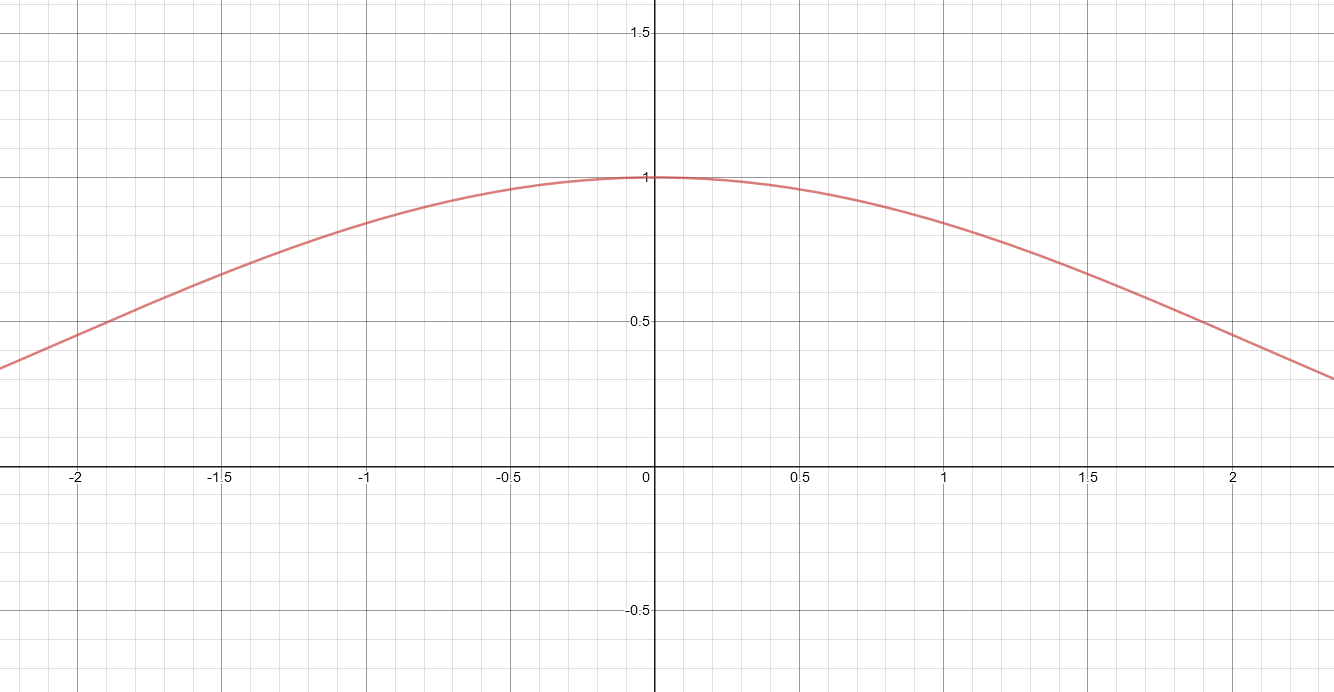We can use L’Hôpital’s Rule:

$$\lim_{x \rightarrow 0}\frac{sinx}{x} =\lim_{x \rightarrow 0}\frac{\frac{d}{dx}(sin x)}{\frac{d}{dx}(x)}=\lim_{x \rightarrow 0}\frac{cosx}{1} =cos 0=1$$

Another option is to use the squeeze theorem. This graph shows that $$\frac{sin x}{x}$$ is between the cosine function and the horizontal line y=1.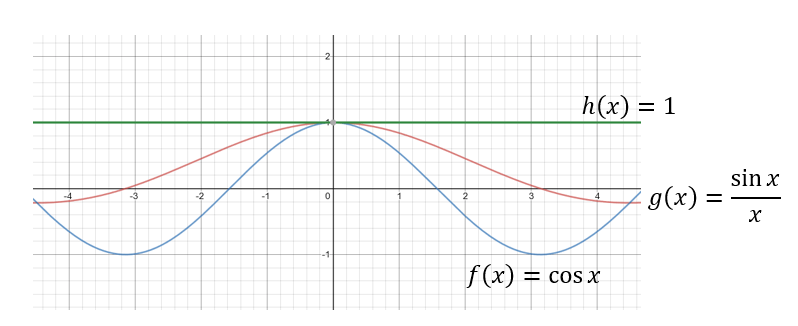When looking for the “between-ness”, we’ll zoom in close to x = 0.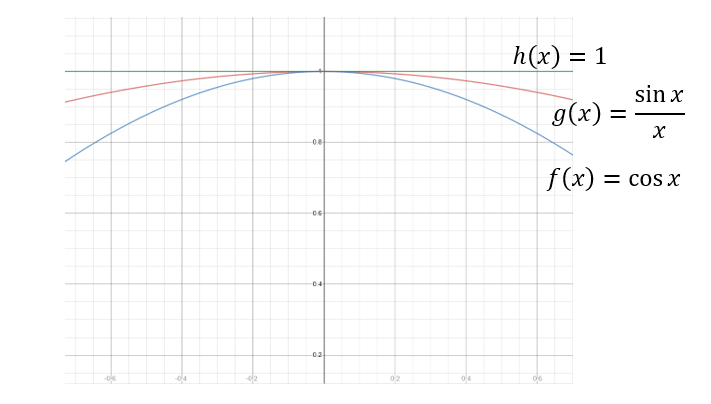No matter how close we zoom in to the graph, $$\frac{sin x}{x}$$ will always be between cos x and 1. By between, we are referring to the function outputs. Let’s zoom in numerically. Of course we can’t use 0, but we can use numbers really close to 0:

 x -0.1 -0.01 0.01 0.1 h(x)=1 1 1 1 1 $$g(x)=\frac{sin x}{x}$$ 0.998 0.99998 0.99998 0.998 f(x)=cos x 0.995 0.99995 0.99995 0.995

No matter how close to 0 we get, the values of g(x) are always between the values of f(x) and h(x).

So what does this do for us? Well, since we know these two things, we automatically know $$\lim_{x \rightarrow 0}\frac{sin x}{x}=1$$

• $$\frac{sin x}{x}$$ is between cos x and 1 around x = 0,
• $$\lim_{x \rightarrow 0}cos x=\lim_{x \rightarrow 0}1=1$$

$$\frac{sin x}{x}$$ is squeezed between cos x and 1 around x=0. Since cos x and 1 both approach 1 as x approaches 0, then $$\frac{sin x}{x}$$ must as well. It’s sort of an indirect way of finding a limit and it’s easy to see why names like squeeze, pinch, sandwich and police are used to describe the theorem.

Now for the formal statement:

If $$f(x)≤g(x)≤h(x)$$ and $$\lim_{x \rightarrow a}f(x)=\lim_{x \rightarrow a}h(x)=L$$, then $$\lim_{x \rightarrow a}g(x)=L$$.

Using the theorem often involves more of a proof-like approach than a method and there are often many ways to use it to prove the value of a limit. For example, with the previous limit, we didn’t need to use the functions cos x and 1 as upper and lower bounds. We could have used $$x^2+1$$ and $$-x^2+1$$ since they both approach 1 as x approaches 0 and $$\frac{sin x}{x}$$ is between them: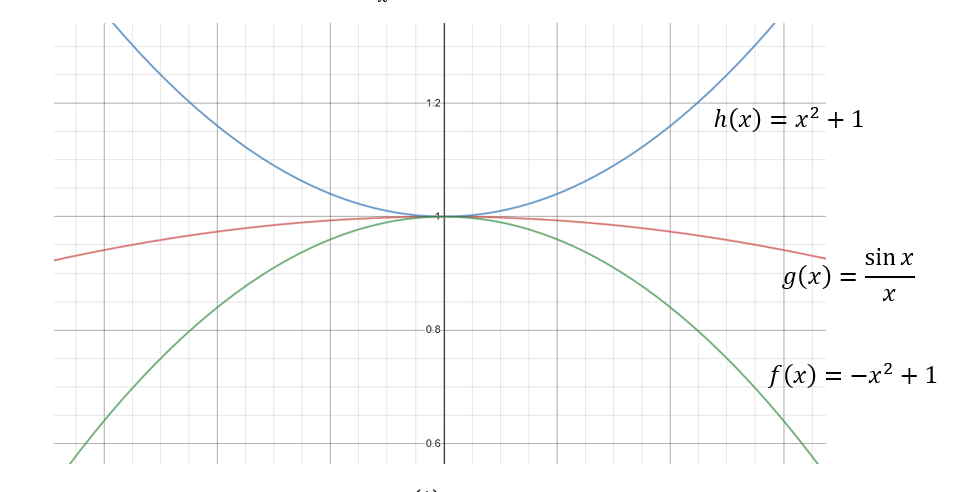Let’s use the squeeze theorem to find $$\lim_{x \rightarrow 0}x^2cos(\frac{1}{x})$$. The table and graph show the function oscillates around the x-axis, but the limit is 0: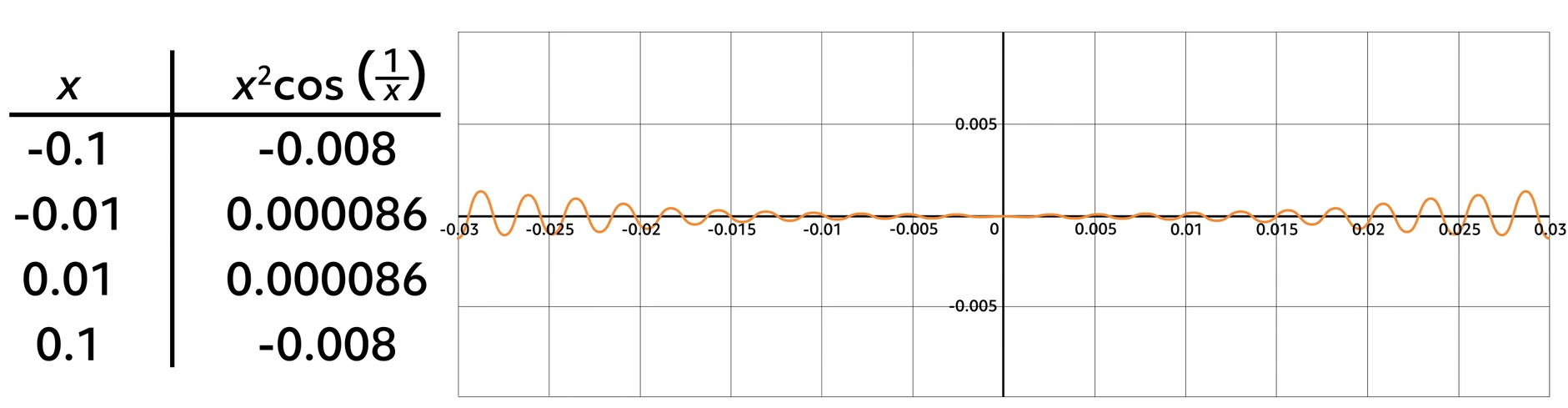Using substitution and algebra is fruitless, so we’ll begin with the $$cos (\frac{1}{x}$$ piece, because that x in the denominator is what’s really complicating things.

Based on our knowledge of the cosine function, we know:

$$-1≤cos (\frac{1}{x}) ≤1$$

Now multiply all three parts of the sentence by $$x^2$$, because the result will be the original function:

$$-x^2≤x^2 cos \frac{1}{x}≤ x^2$$

This tells us how to squeeze the function: put it between $$-x^2$$ and $$x^2$$. Let’s take a look.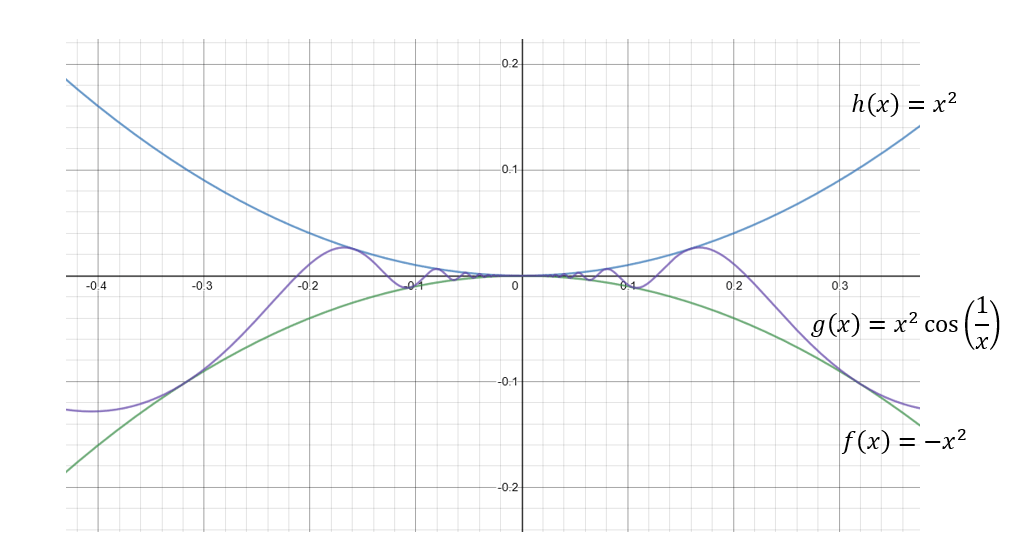According to the theorem, since $$\lim_{x \rightarrow 0}x^2=\lim_{x \rightarrow 0} -x^2 =0$$ and $$x^2cos (\frac{1}{x}$$ is between $$x^2$$ and $$-x^2$$, $$\lim_{x \rightarrow 0}x^2cos (\frac{1}{x})=0$$.

Suppose $$f(t)=-\frac{2}{3}t^3+t^2+\frac{1}{3}$$ and $$h(t)=cos \frac{tπ}{2}$$. If f(t)≤g(t)≤h(t), use the squeeze theorem to show that $$\lim_{t \rightarrow 2}g(t)=-1$$

Now, we don’t know what g(t) is, but with the squeeze theorem we can prove this limit. All we need to show is the limits of f(t) and h(t) are the same as x approaches 2. Since we know that g(t) is pinched in between them, if those limits match, we’ll have our limit.

$$\lim_{t \rightarrow 2}f(t)=-\frac{16}{3}+4-\frac{1}{3}=-1$$ and $$\lim_{t \rightarrow 2}h(t)=cos(π)=-1$$.

Since the limits of f(t) and h(t) are both -1 and they are squeezing g(t),$$\lim_{t \rightarrow 2}g(1)=-1$$.

Let’s try one more!

If $$-\frac{x^2}{4}-\frac{x}{2}≤f(x)≤\sqrt{-\frac{1}{9x}}$$, use the squeeze theorem to find $$\lim_{x \rightarrow -1}f(x)$$ .
$$\lim_{x \rightarrow -1}-\frac{x^2}{4}-\frac{x}{2}=-\frac{1}{4}+\frac{1}{2}=\frac{1}{4}$$
$$\lim_{t \rightarrow -1}\sqrt{-\frac{1}{9x}}=\sqrt{\frac{1}{9}}=\frac{1}{3}$$

In this case, f(x) is between the two functions, but it’s not being squeezed. Since their limits as x approaches -1 do not match, the best we can say is that $$\lim_{t \rightarrow -1}f(x)$$ is between $$\frac{1}{4}$$ and $$\frac{1}{3}$$.

Thanks for squeezing a little time out of your busy schedule to explore this theorem! I hope this video helped you understand when it’s needed and how it works!

See you next time!

## Practice Questions

Question #1:

Which of the following sets of functions $$f\left(x\right)$$ and $$h\left(x\right)$$ (denoted with red lines) would be most appropriate to use to apply the squeeze theorem and find the $$g(x)$$ (where $$g\left(x\right)$$ is shown as a blue line)?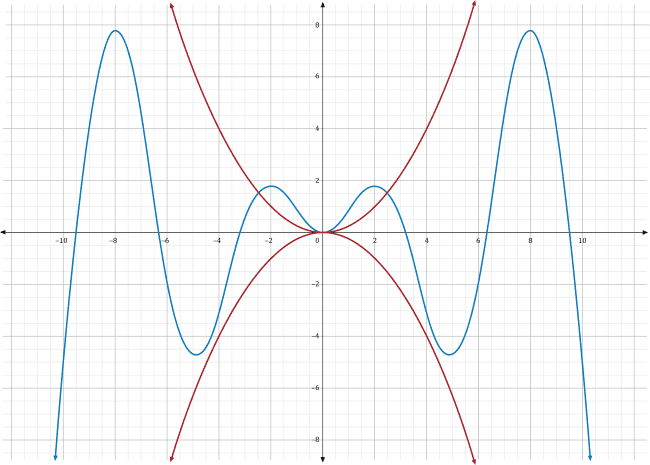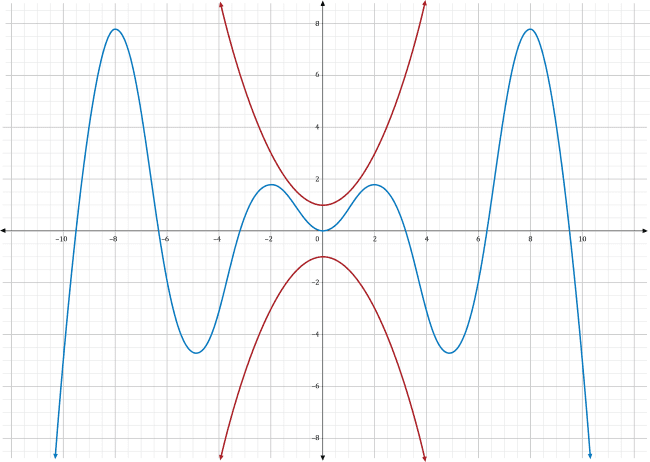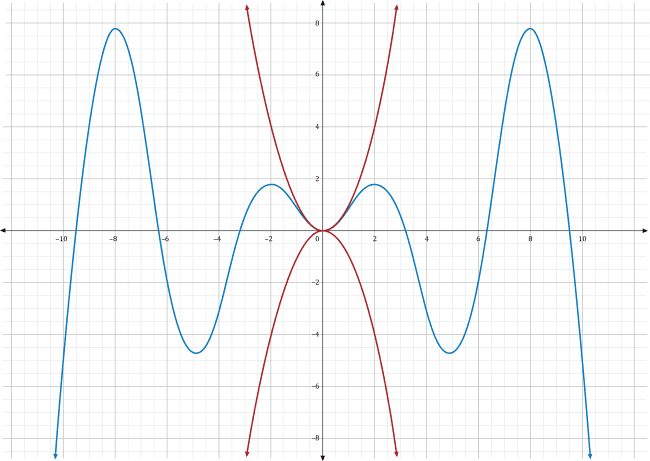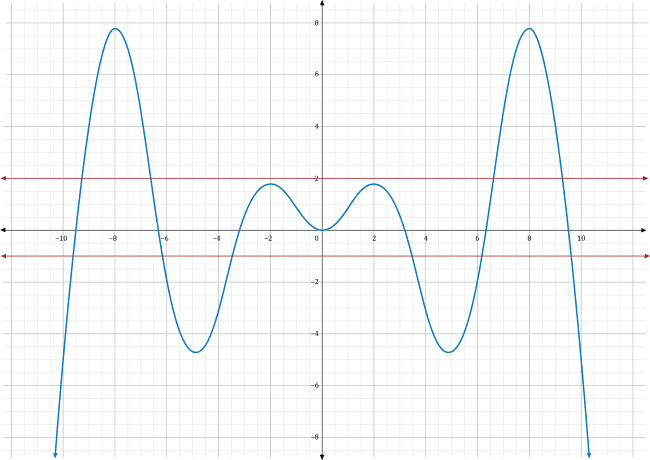The squeeze theorem states that if $$f\left(x\right)$$, $$g\left(x\right)$$, and $$h\left(x\right)$$ are functions such that $$f\left(x\right)\le g\left(x\right)\le h\left(x\right)$$ and $$lim_{x\rightarrow a}\ f\left(x\right)=lim_{x\rightarrow a}\ h\left(x\right)=L$$, then the limit $$lim_{x\rightarrow a}g\left(x\right)=L$$. In other words, one way to find the limit of a function $$g\left(x\right)$$ as $$x$$ approaches some point $$a$$ is by finding two functions $$f\left(x\right)$$ and $$h\left(x\right)$$ that enclose $$g\left(x\right)$$. If $$f\left(x\right)$$ and $$h\left(x\right)$$ come to the same limit $$L$$ as $$x$$ approaches $$a$$, then the $$g(x)$$ must equal $$L$$ too. Notice the two conditions of the squeeze theorem: the functions $$f\left(x\right)$$ and $$h\left(x\right)$$ need to be above and below $$g\left(x\right)$$, respectively, and they both need to come to the same limit value.

In choice A, the two red functions both fall below $$g\left(x\right)$$ in the area near $$x=0$$. Since the limit is taken in that region, these two red functions are not usable for the squeeze theorem.

Choice B depicts two red functions which are fully above and fully below $$g\left(x\right)$$, including the area near $$x=0$$. However, they approach two different $$y$$-values as $$x$$ approaches zero. The top function approaches $$y=1$$ while the bottom function approaches $$y=-1$$. These two functions will not allow you to find the exact value of the limit of $$g\left(x\right)$$ as $$x$$ approaches zero.

Choice C shows two red functions which are entirely above and entirely below $$g\left(x\right)$$, respectively. Additionally, as $$x$$ approaches zero, they both approach the same value, $$y=0$$. For these reasons, the two red functions in choice C are usable for the squeeze theorem. Further, they suggest that the limit $$g\left(x\right)=0$$.

Choice D shows two red functions which are above and below $$g\left(x\right)$$ near $$x=0$$. However, they are approaching different values. The top function is going to 2 while the bottom is going to –1. These two functions will not allow you to find the exact value of the limit of $$g\left(x\right)$$ as $$x$$ approaches zero.

Question #2:

Given that $$f\left(x\right)=-2x^2+12x-18$$, $$h\left(x\right)=\frac{1}{3}x^2-2x+3$$, and $$f\left(x\right)\le g\left(x\right)\le h\left(x\right)$$, use the squeeze theorem to determine $$g(x)$$ .

-2

0

3

The exact value of the limit of $$g\left(x\right)$$ cannot be determined based on the information given.

Even though the problem doesn’t explicitly state the function $$g\left(x\right)$$, the squeeze theorem can help determine the limit of $$g$$ as $$x$$ approaches 3, as long as the two conditions of the theorem are met.

The squeeze theorem says that if $$f\left(x\right)\le g\left(x\right)\le h\left(x\right)$$ and $$f(x)=h(x)=L$$, then the limit $$g(x)$$ is also L. In this problem, the first condition, $$f\left(x\right)\le g\left(x\right)\le h\left(x\right)$$, is given as true. This means to find the limit of $$g\left(x\right)$$ as $$x$$ approaches 3, check if $$f\left(x\right)$$ and $$h\left(x\right)$$ both come to the same limit at that point, and determine the value of those limits.

For this problem, the limits of $$f\left(x\right)$$ and $$h\left(x\right)$$ as $$x$$ approaches 3 can be determined by plugging in $$x=3$$.

$$f\left(x\right)=\left(-2x^2+12x-18\right)$$
$$=-2\left(3\right)^2+12\left(3\right)-18$$
$$=-2\left(9\right)+12\left(3\right)-18$$
$$=-18+36–18$$
$$=0$$

$$h(x)=\left(\frac{1}{3}x^2-2x+3\right)$$
$$=\frac{1}{3}\left(3\right)^2-2\left(3\right)+3$$
$$=\frac{1}{3}\left(9\right)-2\left(3\right)+3$$
$$=3-6+3$$
$$=0$$

So $$f(x)=h(x)=0$$. Since both limits have the same value, zero, the squeeze theorem dictates that the limit of $$g\left(x\right)$$ as $$x$$ approaches 3 must also be zero.

$$g(x)=0$$

Question #3:

Let $$a\left(x\right)=\frac{1}{4}x^2+x+3$$ and $$c\left(x\right)=x^2+4x+6$$. Apply the squeeze theorem to determine the value of $$b(x)$$ given that $$a\left(x\right)\le b\left(x\right)\le c\left(x\right)$$.

0

1

2

3

It is stated that $$b\left(x\right)$$ lies between $$a\left(x\right)$$ and $$c\left(x\right)$$, so all that is needed to apply the squeeze theorem is determining whether $$a$$ and $$c$$ have the same limit as $$x$$ approaches –2. Both of those limits can be found by plugging in $$x=-2$$.

$$a(x)=\frac{1}{4}\left(-2\right)^2+\left(-2\right)+3$$
$$=\frac{1}{4}\left(4\right)-2+3$$
$$=1-2+3$$
$$=2$$
$$c(x)=\left(-2\right)^2+4\left(-2\right)+6$$
$$=4-8+6$$
$$=2$$

Both $$a$$ and $$c$$ have a limit of 2 as $$x$$ approaches –2. Because the function $$b\left(x\right)$$ lies between $$a\left(x\right)$$ and $$c\left(x\right)$$, the squeeze theorem dictates that $$b(x)$$ is also 2.

Question #4:

Carlos is modeling daily average gas prices for the month and determines that the price of gas in dollars for day $$x$$ can be represented by some function $$g\left(x\right)$$ which lies between the curves $$f\left(x\right)=\sqrt{x+1}$$ and $$h\left(x\right)=\frac{1}{6}x+\frac{5}{3}$$. Can the squeeze theorem be applied to determine the limit of $$g\left(x\right)$$ as $$x$$ approaches 8? If so, what is the value of the limit?

No

Yes; $$g(x)=\frac{3}{2}$$

Yes; $$g(x)=3$$

Yes; $$g(x)=\frac{11}{3}$$

The problem states that $$g\left(x\right)$$ is a function lying between $$f\left(x\right)$$ and $$h\left(x\right)$$. So, the first condition of the squeeze theorem is met. The only thing necessary to check is if $$f$$ and $$h$$ come to the same limit as $$x$$ approaches 8. This can be checked by substituting $$x=8$$ into each function.

$$f(x)=\sqrt{8+1}=\sqrt9=\pm 3$$

Since we are talking about gas prices, only use the positive value for this limit.

$$h(x)=\frac{1}{6}\left(8\right)+\frac{5}{3}=\frac{8}{6}+\frac{5}{3}=\frac{4}{3}+\frac{5}{3}=\frac{9}{3}=3$$

It is now clear that $$f$$ and $$h$$ do come to the same limit as $$x$$ approaches 8. Therefore, the squeeze theorem can be applied to find the value of $$g(x)$$. That limit is the same as $$f(x)$$ and $$h(x)$$, which is 3.

Question #5:

Eloise is working to determine the effectiveness of her company’s new marketing strategies by plotting revenue by day, where $$x$$ represents the days since the new strategies were implemented. She determines that the revenue function $$r\left(x\right)$$ is bounded below by the function $$p\left(x\right)=30x+5{,}000$$, and bounded above by the function $$q\left(x\right)=\frac{3}{4}x^2+5{,}300$$. What is the value of $$r(x)$$?

$$r(x)=5{,}600$$
$$r(x)=5{,}900$$
$$r(x)=6{,}300$$
$$r(x)=6{,}550$$

To use the squeeze theorem, $$r\left(x\right)$$ must lie between $$p\left(x\right)$$ and $$q\left(x\right)$$, and $$p$$ and $$q$$ must have the same limit as $$x$$ approaches 20. In this problem, it is given that $$r$$ is between $$p$$ and $$q$$ when the problem states that $$r$$ is bounded below by $$p$$ and bounded above by $$q$$. This means the only remaining condition is checking if $$p$$ and $$q$$ have the same limit as $$x$$ approaches 20. This can be done by substituting $$x=20$$ into both functions.

$$p(x)=30\left(20\right)+5{,}000=600+5{,}000=5{,}600$$
$$q(x)=\frac{3}{4}\left(20\right)^2+5{,}300=\frac{3}{4}\left(400\right)+5{,}300=300+5{,}300=5{,}600$$

Because $$p$$ and $$q$$ have the same limit as $$x$$ approaches 20, and because $$r$$ lies between them, the squeeze theorem dictates that $$r(x)=5{,}600$$.